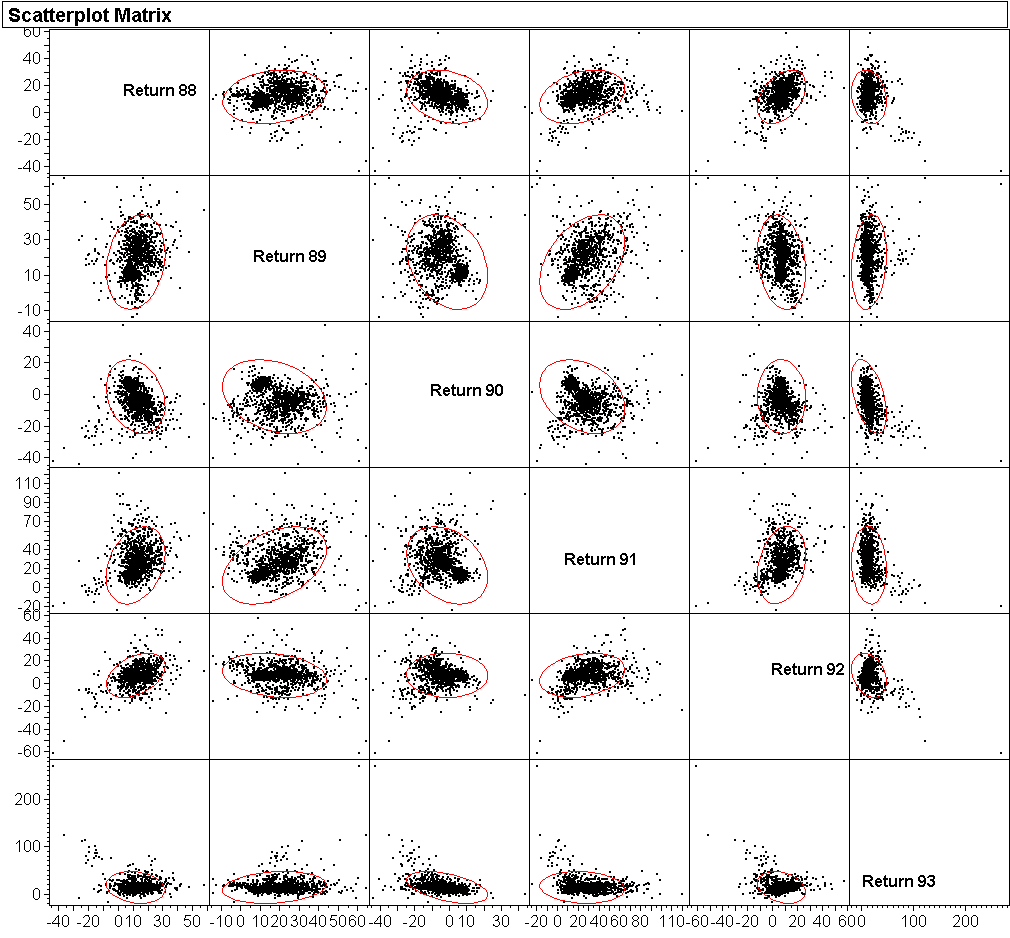## Plotting Techniques, STAT 603

This page summarizes the types of plots we have encountered in the pre-term STATS 603 class. Here is a list of plot types: histograms, boxplots, normal quantile plots, bar charts, spine plots, scatterplots, comparison boxplots, mosaic plots, time series plots, control charts. We will bring some order to this collection by showing when a particular plot is useful, what can be learned from it, and how it is generated in JMP.

[In what follows we will use the terms "variable" and "column" interchangeably.]

PLOT TYPES are largely determined by two factors:

• NUMBER OF VARIABLES:

• MODELING TYPE OF THE VARIABLES:
• Continuous C: Measurements and counts. The values must be numbers and are interpreted as such. They can be integers, though, and insofar not very "continuous".
• Ordinal/nominal O/N: Group labels. The values can be strings or numbers, but they are interpreted as labels of groups. For ordinal variables the groups have an order; for nominal variables they don't (O: Jan, Feb, Mar,...; N: brown, pink, purple,...).
How JMP thinks: It doesn't want to hear "scatterplot". It wants you to ask for a bivariate plot ("Fit Y by X"), and if you choose variables that are both continuous, it will indeed give you a scatterplot. If, however, both variables are nominal or ordinal, it will give you a mosaic plot. Similarly, you don't ask for a histogram. You ask for a univariate plot ("Distribution"), and if the variable you choose is continuous, you get a histogram, otherwise a barchart. In other words, JMP decides for you what bivariate or univariate plot makes sense.

[Problem: Sometimes you get the wrong type of plot, such as when the modeling type of an integer variable is continuous, but you really want to know how often each integer occurs. You can't tell JMP to hand you a barchart instead of a histogram, or a mosaic plot instead of a scatterplot. Instead, you have to change the modeling type from continuous to ordinal: R-click on column name > Column Info > Modeling Type > ordinal. Btw, variables with character data cannot be made continuous!]

### UNIVARIATE PLOTS

With univariate plots you examine the distribution of one variable at a time, not the associations and dependences between two or more variables.

JMP: Analyze > Distribution; you can now specify as many variables as you please by selecting them as Y-variables. You will get one plot for each variable separately.

Rule: Make univariate plots of ALL variables first thing when you start looking at a new dataset.

Here are the univariate plot types as a function of the modeling types:

• CONTINUOUS UNIVARIATE PLOTS:

For continuous variables, JMP gives you a vertical histogram and a boxplot on the same scale. You can then ask for a normal quantile plot, which will be attached to the boxplot, so that all three plots (histogram, boxplot, normal quantile plot) share the vertical scale of the data.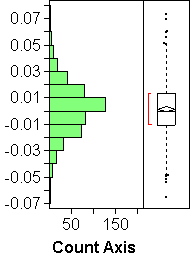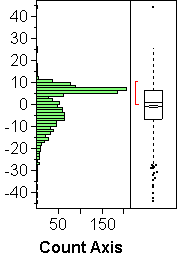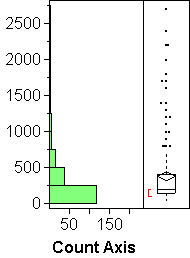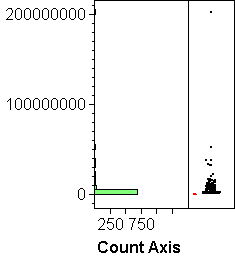(a) (b) (c) (d)

• HISTOGRAMS:
The bars represent the frequency of observations in the intervals. A histogram shows the overall shape of the distribution of a variable: where is lots, where little. Things we can see well in a histogram:
• MODES or humps or peaks of the distribution. In histogram (b) above we see two modes. The vertical axis represents returns of 1529 mutual funds in 1990; the upper mode shows essentially the bond funds that out-performed the much more volatile stock funds of the lower mode in that year.
• SKEWNESS or asymmetry in the tails; one tail of the distribution is more spread out than the other. Skewness occurs often when the values are bounded on one side, such as zero. See histogram (c) above; histogram (d) is an extreme case of skewness.
• NORMALITY (not really): If a histogram has only one mode, looks roughly symmetric, and doesn't have outliers, it may be an indication of an approximately normal distribution, as in histogram (a) above. But histograms are NOT the best way to check normality! Use normal quantile plots instead.

• BOXPLOTS:
Boxplots summarize mostly information contained in quantiles:
1. The box represents the half of the data between the lower and upper quartiles. Its height is therefore the IQR (inter-quartile range).
2. The line in the middle shows the median.
3. The diamond represents the mean and its standard error.
4. The whiskers are drawn from each end of the boxes to a length of 1.5*IQR or the min or max, whichever comes first.
5. Extreme observations outside the whiskers are shown individually, and they are thought of as potential outliers.
Things we can see well with boxplots are therefore:
• OUTLIERS: The points outside he whiskers may be outliers (or not). In boxplot (c) above, the whole upper mode is shown as potential outliers because the lower mode near zero contains 3/4 of the data and hence determines the box single-handedly.
• SKEWNESS: A notorious indicator of skewness is a large discrepancy between the mean and the median. See boxplots (b) and (c) above; boxplot (d) is so ridiculously skewed that the box and the mean are both squished into the bottom ink.

• NORMAL QUANTILE PLOTS:
The only -- but important -- purpose of normal quantile plots is to check approximate normality of the distribution of a variable.

JMP: First create a histogram and boxplot (Analyze > Distribution); then click on the red diamond next to variable name > Normal Quantile Plot. This has to be repeated for every histogram/boxplot you wish to augment.

Description: JMP's default normal quantile plot has on the vertical axis the values of the variable, on the same scale as the adjacent boxplot and histogram. On the horizontal axis are the theoretical quantiles of the standard normal distribution N(0,1). If the variable is distributed according to a normal distribution N(mu,sigma^2) (with arbitrary mu and sigma), then the dots will be near a straight line. The reason is that the observed quantiles of the variable (its median, quartiles, quintiles,...) will line up with the corresponding quantiles of the standard normal distribution according to the equation y = mu + sigma*x, up to sampling variation.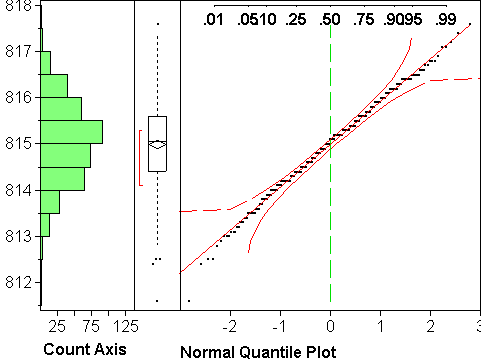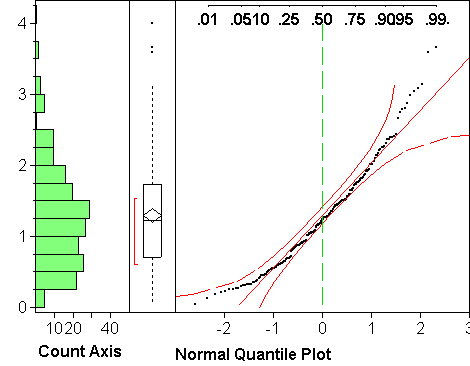(a) (b)

Interpretation: In those places where the points are close to the straight line, the theoretical and observed quantiles are in good agreement; wherever the points are substantially off the straight line, the agreement is unsatisfactory. In other words, the plot shows where the quantiles of the estimated normal distribution N(m,s^2) make a good approximation to the observed quantiles. If the agreement is good everywhere, one can replace the observed quantiles with those of the estimated normal distribution, which can be useful when estimating probabilities P(Y<c). Note that being (say) above the straight line means opposite things on either end: on the low end, it means the tail of the distribution is too short/light to be normal, on the upper end it means the tail is too long/heavy to be normal (think about it).

Assessment: The remaining question is how to tell good agreement. To this end, JMP provides two curves, one above, one below the straight line. If the points wander outside the area between the two curves, it is evidence against a normal distribution, at least in those areas. The curves could be closer to the line and still be valid, so stepping outside is indeed strong evidence against normality, while staying inside is not sufficient evidence for normality. Note that the area between the curves widens on both ends. Reason: Extreme quantiles are less reliably estimated than quantiles in the center. Extreme quantiles can vary more wildly without being evidence against a normal distribution.

Examples: Plot (a) above looks very normal. It's main failing is the staircasing, which stems from the rounding of the values to multiples of 0.1. Plot (b) shows upward or right skewness: The upper end deviates upwards from the line, and so does the lower end, which means according to the above that the upper tail of the distribution is too heavy, the lower tail too light. Points do not move outside the curves on either end, though, which might make us think the deviations are not significant. Skewness is clearly there, though, both in the histogram and the normal quantile plot. This example illustrates the fact that the curves are wider than they should be.

• ORDINAL/NOMINAL UNIVARIATE PLOTS:

For ordinal and nominal variables, JMP gives you a vertical barchart and a spineplot on the same scale. Btw, JMP does not use the terms "barchart" and "spineplot"; instead, barcharts appear in menus as "Histogram", and spineplots as "Mosaic Plot". We prefer to reserve the term "histogram" for continuous variables and the term "mosaic plot" for bivariate frequency plots.

Besides the obvious use for frequency comparisons of groups, these plots have a particular use in data cleaning: it often happens that misspellings of group labels lead to the spurious multiplication of groups, as when the group labels should be "YES" and "NO", but some were coded as "Yes" or "no". A barchart or spine plot will quickly identify the problem.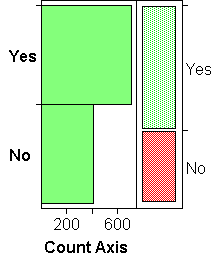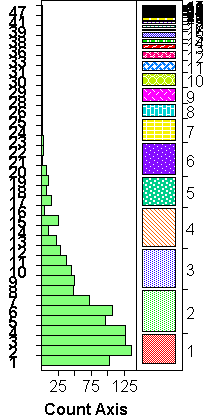(a) (b) (c)

The examples above show barcharts and spineplots for ordinal/nominal variables with 2, 4, and many, groups.

• BARCHARTS:
Barcharts are similar to histograms, except that there is no choice of binwidth: the bins are the groups defined by the ordinal or nominal variable. The height of a bar is proportional to the frequency of cases in the group. For ordinal variables, groups are shown in order; for nominal variables, group ordering depends on the "Data Type" (R-click on column label > Col Info): groups are ordered lexicographically if the data type is "Character", and numerically if it is "Numeric".

• SPINEPLOTS:
Spineplots convey the same information as a barcharts, just less well so: spine plots code frequency by the width, barcharts by the height of the bars. (Note, though, that the width is vertical, just as the height of the bars in barcharts is horizontal, due to JMP's vertical default arrangement).

### MULTIVARIATE PLOTS: SCATTERPLOT MATRICES

A scatterplot matrix is the collection of all possible pairwise scatterplots of a set of continuous variables. They are created by "Analyze > Multivariate" and picking a number of continuous variables as Y's.

Scatterplot matrices take some getting-used-to because of the way the axes are labeled. The example below illustrates the conventions: In the first column, McDonald is the horizontal axis; in the second column it is Disney;... Analogously, in the first row McDonalds is the vertical axis; in the second row it is Disney;... Each pair of variables is shown twice. For example, Disney vs McDonalds (Y vs X) is the first plot in the second row. The reverse, McDonalds vs Disney is the second plot in the first row.

The plots are augmented with ellipses that cover 95% of the data. The narrower the ellipse, the stronger the linear association (correlation) between the two variables. We see, for example, that McDonalds and Disney has the highest correlation among the three possible pairs.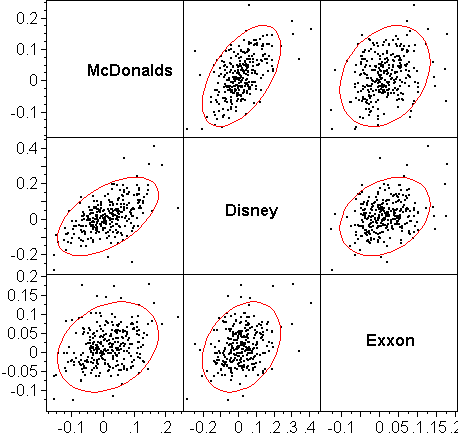The following example illustrates the power but also the need for real estate for scatterplot matrices. We are shown plots of returns, year vs year, from 1988 to 1993, for 1529 mutual funds. Because much of the interesting structure (clustering, outliers) is not of the type of linear association, the ellipses are mostly not useful; they could have been removed.